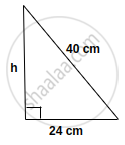# Find the area of the right-angled triangle with a hypotenuse of 40 cm and one of the other two sides of 24 cm. - Mathematics

Sum

Find the area of the right-angled triangle with a hypotenuse of 40 cm and one of the other two sides of 24 cm.

#### Solutionby applying Pythagoras' theorem,

H2 = P2 + B2

402 = P2 + 242

P2 = 402 - 242

= (40 - 24) (40 + 24)

= 16 × 64 = 1024 cm2

P = sqrt1024 = 32 cm

A = 1/2 xx  "b" xx "h"

= 1/2 xx 24 xx 32

= 12 xx 32

= 384 cm2

Is there an error in this question or solution?
Chapter 20: Area of a Trapezium and a Polygon - Exercise 20 (A) [Page 224]

#### APPEARS IN

Selina Concise Mathematics Class 8 ICSE
Chapter 20 Area of a Trapezium and a Polygon
Exercise 20 (A) | Q 13 | Page 224
Share